# Understanding of OpenSeq2Seq

Prerequisites: LTSM, GRU

In this article, we will be discussing a deep learning toolkit used to improve the training time of the current Speech Recognition models among other things like Natural Language Translation, Speech Synthesis and Language Modeling. Models built using this toolkit give a state-of-the-art performance at 1.5-3x faster training time.

OpenSeq2Seq

OpenSeq2Seq is an open-source TensorFlow based toolkit featuring multi-GPU and mixed-precision training which significantly reduces the training time of various NLP models. For example,

```1. Natural Language Translation: GNMT, Transformer, ConvS2S
2. Speech Recognition: Wave2Letter, DeepSpeech2
3. Speech Synthesis: Tacotron 2
```

It uses Sequence to Sequence paradigm to construct and train models to perform a variety of tasks such as machine translation, text summarization.

Sequence to Sequence model

The model consists of 3 parts: encoder, encoder vector and decoder.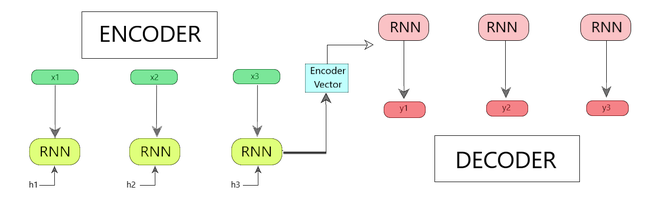Fig 1 : Encoder-Decoder Sequence to Sequence Model

• Encoder
• In this, several recurrent units like LTSM (Long Short Term Memory) and GRU (Gated Recurrent Unit) are used for enhanced performance.
• Each of these recurrent units accepts a single element of the input sequence, gathers the information for that element and propagates it forward.
• The input sequence is a collection of all the words from the question.
• The hidden states (h1, h2…, hn) are calculated using the following formula. [Eq 1]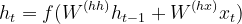Eq 1

```where,
ht = hidden state
ht-1 = previous hidden state
W(hh) = weights attached to the previous hidden state. (ht-1)
xt = input vector
W(hx) = weights attached to the input vector.

```
• Encoder Vector
• The final hidden state is calculated using Eqn 1 from the encoder part of the model.
• The encoder vector collects the information for all input elements in order to help the decoder make accurate predictions.
• It serves as the initial hidden state of the decoder part of the model.

• Decoder
• In this, several recurrent units are present where each one predicts an output yt at a time step t.
• Each recurrent unit accepts a hidden state from the previous unit and produces an output as well as its own hidden state.
• The hidden states (h1, h2…, hn) are calculated using the following formula. [Eqn 2]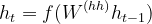Eqn 2

For example, Fig 1. Shows sequence to sequence model for a dialogue system.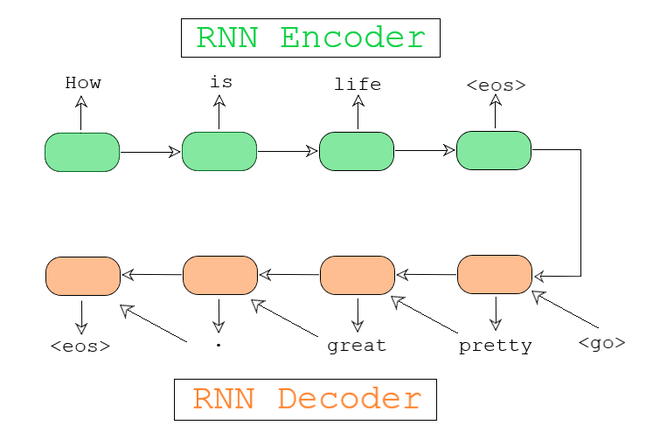Fig 2 : Sequence to Sequence model for a Dialog System

Every Sequence to Sequence model has an encoder and a decoder. For example,

Design and Architecture

The OpenSeq2Seq toolkit provides various classes from the user can inherit their own modules. The model is divided into 5 different parts :

1. Data Layer
2. Encoder
3. Decoder
4. Loss Function
5. Hyperparameters
• Optimizer
• Learning Rate
• Dropout
• Regularization
• Batch_Size etc.
```For example, an OpenSeq2Seq model for Machine Translation would look like :

Encoder - GNMTLikeEncoderWithEmbedding
Decoder - RNNDecoderWithAttention
Loss Function - BasicSequenceLoss
Hyperparameters -
Learning Rate = 0.0008
Regularization = 'weight decay'
Batch_Size = 32
```

Mixed-Precision Training:

When using float16 to train large neural network models, it is sometimes necessary to apply certain algorithmic techniques and keep some outputs in float32. (hence the name, mixed precision).

Mixed-Precision Support [using Algorithm]

The model uses TensorFlow as its base, thus have tensor-cores which delivers the required performance to train large neural networks. They allow matrix-matrix multiplication to be done in 2 ways:

• Single-Precision Floating-Point (FP-32)
• A  single-precision floating-point format is a computer number format that occupies 32 bits (four bytes in modern computers)  in computer memory.
• In a 32-bit floating-point, 8 bits are reserved for the exponent (“magnitude”) and 23 bits for the mantissa (“precision”).

• Half-Precision Floating Point (FP-16)
• A half precision is a binary floating-point format is a computer number format that occupies 16 bits (two bytes in modern computers) in computer memory.

Earlier, when training a neural network, FP-32 (as shown in Fig 2) were used to represent the weights in the network because of various reasons such as:

• Higher Precision — 32-bit floats have enough precision such that we can distinguish numbers of varying magnitudes from one another.

• Extensive Range — 32-bit floating points have enough range to represent numbers of magnitude both smaller (10^-45) and larger (10^38) than what is required for most applications.

• Supportable — All hardware (GPUs, CPUs) and APIs support 32-bit floating-point instructions quite efficiently.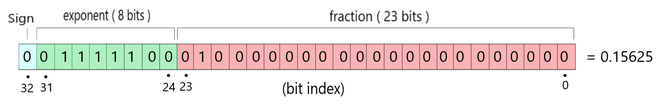Fig 3: FP-32 representation

But, later on, it was found that for maximum deep learning models, so much magnitude and precision is not required. So, NVIDIA created hardware that supported 16-bit floating-point instructions and observed that most weights and gradients tend to fall well within the 16-bit representable range.

Therefore, in OpenSeq2Seq model, FP-16 has been used.  Using this, we effectively prevent wasting all those extra bits. With FP-16, we reduce the number of bits in half, reducing the exponent from 8 bits to 5, and the mantissa from 23 bits to 10. (As shown in Fig 3)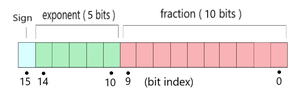Fig 4: FP-16 representation

Risks of using FP-16

1. Underflow : attempting to represent numbers so small they clamp to zero.
2. Overflow : numbers so large (outside FP-16 range) that they become NaN, not a number.

• With underflow, our network never learns anything.
• With overflow, it learns garbage.
• For Mixed-Precision Training, we follow an algorithm that involves the following 2 steps:
```Step 1 - Maintain float32 master copy of weights for weights update while using the float16
weights for forward and back propagation.
Step 2 - Apply loss scaling while computing gradients to prevent underflow during backpropagation.
```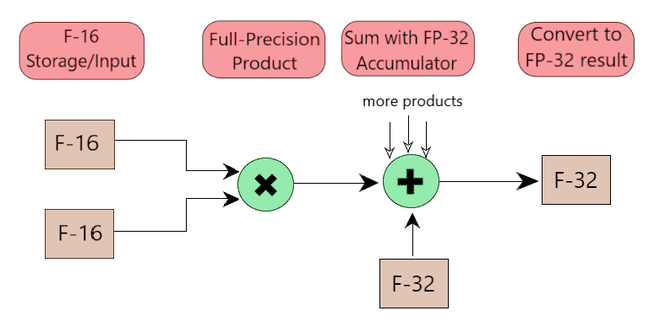Fig 5: Arithmetic Operations in FP16 and accumulated in FP32

The Mixed Precision Training of the OpenSeq2Seq model involves three things:

1. Mixed Precision Optimizer
2. Mixed Precision Regularizer
3. Automatic Loss Scaling

1. Mixed Precision Optimizer

The model has all variables and gradients as FP-16 by default, as shown in Fig 6. The following steps take place in this process: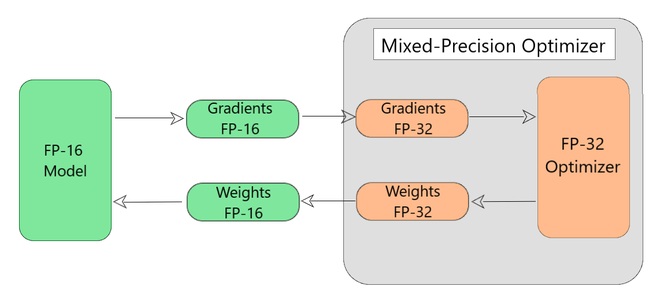Fig 6:  Mixed-precision wrapper around TensorFlow optimizers

```Working of Mixed Precision Wrapper (Step by Step)

Each Iteration
{
Step 1 - The wrapper automatically converts FP-16 gradients and FP-32 and feed them
to the tensorflow optimizer.
Step 2 - The tensorflow optimizer then updates the copy of weights in FP-32.
Step 3 - The updated FP-32 weights are then converted back to FP-16.
Step 4 - The FP-16 weights are then used by the model for the next iteration.
}
```

2. Mixed Precision Regularization

As discussed earlier the risks involved with using F-16 like numerical overflow/underflow. The mixed precision regularization ensures that such cases do not occur during the training. So, to overcome such problems, we do the following steps:

```Step 1 - All regularizers should be defined during variable creation.

Step 2 - The regularizer function should be wrapped with the 'Mixed Precision Wrapper'. This takes care of 2 things:
2.1 - Adds the regularized variables to a tensorflow collection.
2.2 - Disables the underlying regularization function for FP-16 copy.

Step 3 - This collection is then retrieved by Mixed Precision Optimizer Wrapper.

Step 4 - The corresponding functions obtained from the MPO wrapper will be applied to the FP-32 copy
of the weights ensuring that their gradients always stay in full precision.
```

3. Automatic Loss Scaling

The OpenSeq2Seq model involves automatic loss scaling. So, the user does not have to select the loss value manually. The optimizer analyzes the gradients after each iteration and updates the loss value for the next iteration.

Models Involved

OpenSeq2Seq currently offers a full implementation of a variety of models:

OpenSeq2Seq features a variety of models for language modelling, machine translation, speech synthesis, speech recognition, sentiment analysis, and more to come. It aims to offer a rich library of commonly used encoders and decoders. This was a basic overview of the OpenSeq2Seq model covering the intuition, architecture and concepts involved. For any doubts/queries, comment below.

Attention geek! Strengthen your foundations with the Python Programming Foundation Course and learn the basics.

To begin with, your interview preparations Enhance your Data Structures concepts with the Python DS Course.

My Personal Notes arrow_drop_upCheck out this Author's contributed articles.

If you like GeeksforGeeks and would like to contribute, you can also write an article using contribute.geeksforgeeks.org or mail your article to contribute@geeksforgeeks.org. See your article appearing on the GeeksforGeeks main page and help other Geeks.

Please Improve this article if you find anything incorrect by clicking on the "Improve Article" button below.

Article Tags :
Practice Tags :

Be the First to upvote.

Please write to us at contribute@geeksforgeeks.org to report any issue with the above content.